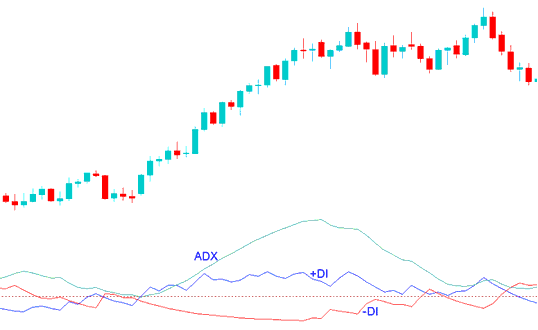# ADX Momentum Indices Breakout Indicator Technical Analysis and DX Momentum Trading Strategies Signals

ADX Momentum Breakout Indicator is used to measure the strength of indices market trends on a scale of 0 - 100; the higher the ADX Momentum value the stronger the current stock indexes trend. It should be noted that while the direction of the stock indexes price is important to the ADX Momentum indicator calculation, the ADX Momentum indicator itself is not a directional indices indicator. ADX Momentum Indices Indicator values above 30 indicate a very strong indices trending market, while ADX Momentum Indices Indicator values below 20 indicate non-trending indices market or a ranging stock indexes market.

Many traders interpret ADX momentum indicator levels turning up from below 20 as an early signal of a new emerging indices trend breakout signal - while declining ADX indicator levels turning down from above 30 as a reversal of the current stock indexes trend.

ADX Momentum indicator should be used as part of a indices trading system that includes the +DI and -DI lines. Most indices trading platforms integrate the original ADX Indices Indicator with the +DI and -DI indicators -DMI Index and collectively refer to the whole indices indicator system as the ADX Momentum Indices Technical Indicator.

In the ADX indices indicator example explained and illustrated below:

ADX Momentum Indices Indicator is the light green line

+DI is the blue line - bullish line

- DI is the red line - bearish lineBullish Indices Trend - when the +DI, blue line is above the -DI, red line

Bearish Indices Trend - when the -DI, red line is above the +DI, blue line

A bullish stock indices signal is generated when the +DI line is above the -DI line, when the blue line is above the red line.

To identify a strong bullish ADX Momentum Breakout signal we wait until the ADX Momentum indicator values move above 20, and the blue line is above the red line. This is a bullish ADX Momentum Breakout signal because the DMI system identifies the indices signal as a buy, while ADX Momentum indicator levels above 20 shows there is a strong upward momentum stock indexes trend.

A bearish signal is generated when the red line is above the blue line i.e. The -DI line is above the +DI line. A strong short signal will be generated when this bearish signal is accompanied by an ADX Momentum value of above 20.

To identify a strong bearish ADX Momentum Breakout signal we wait until the ADX Momentum indicator values move above 20, and the red line is above the blue line. This is a bearish ADX Momentum Breakout signal because the DMI system identifies the indices signal as a sell, while ADX Momentum indicator levels above 20 shows there is a strong downward momentum stock indexes trend.